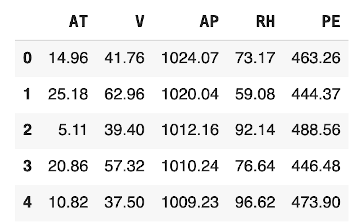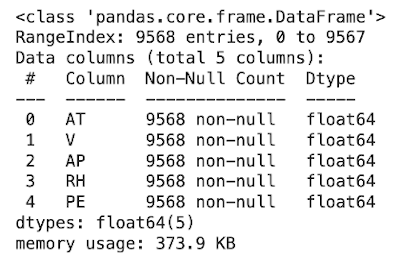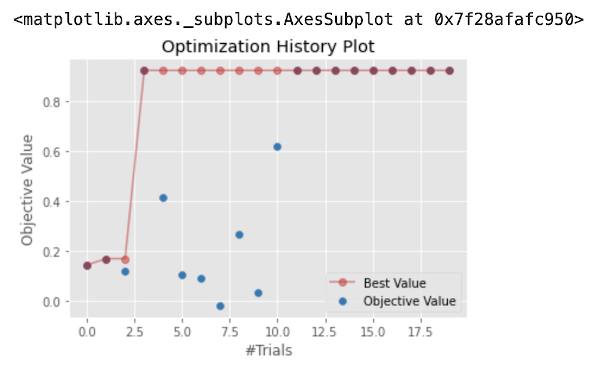| Technical Writer: Thilakraj Devadiga | Technical Review: ABCOM Team | Level: Advanced | Banner Image Source : Internet |

# Motivation

All Machine Learning algorithms use several parameters and getting the appropriate values for these parameters is always an enormous challenge for the ML developers. An in-appropriate value for the parameters results in a lower accuracy for the model. So, how do you fine-tune the values for these parameters? Usually, this is a trial-n-error process, or else you must have a good understanding of the problem domain and the algorithm itself. As this is rare, usually the developers resort to a trial-n-error approach. This can seriously impact your budget on training time and resource utilization during training.

In this tutorial, I will show you how to fine-tune the algorithmic parameters with a readily available automated tool called Optuna.

# Preamble

In my last article, Machine Learning in Insurance Sector, I used the Optuna optimized parameters. I will now show you how I got those parameters. You would then use this technique in all your future hyper-parameter tunings.

The popular sklearn library provides two methods for hyper-parameter tuning. These are called RandomSearchCV and GridSearchCV. Both provide the same functionality except for the fact that the RandomSearchCV as its name specifies selects the parameters from the specified grid at random, while the other one picks them in the specified order. GridSearch is computationally more expensive than RandomSearch when it comes to many parameters.

# What is Optuna?

The Optuna is an automatic hyper-parameter optimization software framework designed for machine learning tasks. It is lightweight, versatile and platform agnostic. It has a simple installation, uses Python syntax for conditions and loops. It adopts state-of-the-art algorithms for sampling hyper-parameters and efficiently pruning unpromising trials. It supports parallelization and provides an excellent visualization for your further investigation of the outputs and studies.

There are two important terms used in Optuna - Study and Trial. Study is the optimization based on an objective function and trial is a single execution of the objective function. The goal of a study is to find out the optimal set of hyper-parameter values through multiple trials.

# Project

I will show you how to fine-tune parameters for the widely used SVM (Support Vector Machines) algorithm. I will use the SupportVectorRegressor of sklearn for the demonstration. We will first implement the baseline model with default parameter values and then implement another one with a few parameters tuned by Optuna. We will compare the accuracies of the two trained models to check the efficiency of Optuna.

For this tutorial I have selected a combined cycle power plant dataset provided in UCI Machine Learning repository. The dataset contains 9568 data points collected from a Combined Cycle Power Plant over 6 years (2006-2011), when the power plant was set to work with full load. A combined cycle power plant (CCPP) is composed of gas turbines (GT), steam turbines (ST) and heat recovery steam generators. In a CCPP, they generate the electricity by gas and steam turbines, which are combined in one cycle, and are transferred from one turbine to another. While the Vacuum influences the Steam Turbine, the other three of the ambient variables affect the GT performance. We have to develop the model to predict the net hourly electrical energy output (PE) based on the features - temperature (T), ambient pressure (AP), relative humidity (RH) and exhaust vacuum (V). The model will use SupportVectorRegressor for predicting the energy output.

# Creating Project

Create a new Colab project and rename it to Optuna Tuning. As we use Optuna in this project, make sure that it is installed in your Colab environment by running the following commands:

``````pip install optuna
``````

Once the Optuna is installed, import the following packages into your project:

``````import pandas as pd
import numpy as np
import seaborn as sns
import warnings
from sklearn.svm import SVR
import optuna as op
from sklearn.model_selection import train_test_split
warnings.filterwarnings("ignore")
``````

Note, we have imported `SVR` from sklearn for our regressor algorithm.

## Dataset

I have uploaded the dataset on our GitHub for your quick access in the Colab project. Load the data into your project using the following command:

``````df=pd.read_csv("https://raw.githubusercontent.com/abcom-mltutorials/Optuna/main/CCPP.csv")
``````

Examine few records of the loaded data:

``````df.head()
``````Before we proceed with the model building, let us check the data.

``````df.info()
``````

This is the output:The function returns details about the dataset. And we can observe that each feature column in the dataset is a continuous value and doesn’t have any null value or missing values. This is great since the dataset requires no extra preprocessing steps.

## Features/Target

As said earlier, our target for model training is the PE column. We will use the rest of the fields as the features.

We extract the features using the following statement:

``````X=df.drop("PE",axis=1)
``````

We create the target variable using dataframe indexing :

``````Y=df.PE
``````

## Training/Testing Datasets

We split the dataset into training and testing using the following statement:

``````X_train,X_test,y_train,y_test=train_test_split(X,Y,test_size=0.2,random_state=123)

print("train: ",X_train.shape)
print("test: ",X_test.shape)
``````

This is the output:

``````train:  (7654, 4)
test:  (1914, 4)
``````

We reserved 20% of data for testing. From the output, we know that the training dataset consists of 7654 data points.

At this point, we are done with data preparation, so let us proceed to model building.

## Model

As I mentioned earlier, we will use the Support Vector Machine algorithm to perform the regression task. Sci-kit learn has the implementation of the SVM Algorithm under its SVM API, from which we require SVR for regression tasks.

First we will create a model with default parameters.

``````model=SVR()
``````

We train the model on our training dataset by calling its `fit` method.

``````model.fit(X_train,y_train)
``````

After the model is trained, we evaluate its performance.

``````model.score(X_test,y_test)
``````

This is the output:

``````0.38922787580732543
``````

We observe that the accuracy is really low for a regression problem and is not acceptable in a real scenario. Now, let's use the Optuna framework to fine-tune the model with a pre-selected set of parameters.

## Selecting Parameters

The SVM algorithm uses several parameters. We will select the following parameters for our algorithm training.

• Kernel - Specifies the kernel type to be used in the algorithm. Possible values are
• linear
• poly
• rbf, and
• sigmoid
• C - Regularization parameter. The strength of the regularization is inversely proportional to C. It must be strictly positive. The penalty is a “squared l2 penalty”.
• Degree - Degree of the polynomial kernel function (‘poly’). Ignored by all other kernels.
• Gamma - Kernel coefficient for ‘rbf’, ‘poly’ and ‘sigmoid’.

## Objective Function

We create a function that is used by the Optuna framework to find the best-values for the parameters.

``````def objective(trial):
kernel=trial.suggest_categorical('kernel',['rbf','poly','linear','sigmoid'])
c=trial.suggest_float("C",0.1,3.0,log=True)
gamma=trial.suggest_categorical('gamma',['auto','scale'])
degree=trial.suggest_int("degree",1,3,log=True)
model =SVR(kernel=kernel,degree=degree,gamma=gamma,C=c)
model.fit(X_train,y_train)
accuracy = model.score(X_test,y_test)
return accuracy
``````

In the above function, we pass a trial object from the Optuna study function. The trial object selects a set of pre-defined values for each parameter that we want to optimize. For example, in the first statement in the function definition, we select the categorical column called kernel with four potential values - rbf, poly, linear, and sigmoid. The second statement sets the values for a float type parameter called C. We set the range of float values here from 1.0 through 3.0. Likewise, we set the values for categorical column gamma to one of the two values - auto and scale. Finally, for the degree column, which is of type int we set the values in the range 1 to 3. After the variables are set, we define the model by passing these variables as parameters.

``````model =SVR(kernel=kernel,degree=degree,gamma=gamma,C=c)
``````

We train the model by calling its `fit` method and return the model’s accuracy to the caller. Optuna runtime will call this objective function multiple times during study, record the accuracy at each iteration and will return you the best accuracy at the end. You decide on the number of iterations - trials. Isn’t it simple?

Optuna provides a method `create_study` to start the above process.

``````study = op.create_study(direction="maximize")
``````

The direction sets the mode for best fit. Use maximize for maximization of the output function and minimize for the minimization. You call the `optimize` method on the study to start the process.

``````study.optimize(objective, n_trials=20,n_jobs=-1)
``````

The n_trials decides the number of iterations and the n_jobs sets parallelization. Each iteration may take a substantial amount of time to complete. Depending on your needs, set this to a proper value. Larger the value, the better would be the results.

When you run the above statement, you would see the following log printed on your console:

``````[I 2021-06-02 03:28:07,286] A new study created in memory with name: no-name-bf2d256e-b5ee-480d-aefe-62b6d88e1851
[I 2021-06-02 03:28:20,369] Trial 1 finished with value: 0.16914260315387564 and parameters: {'kernel': 'rbf', 'C': 0.34174903259097233, 'gamma': 'auto', 'degree': 2}. Best is trial 1 with value: 0.16914260315387564.
[I 2021-06-02 03:28:20,682] Trial 0 finished with value: 0.14451537922876667 and parameters: {'kernel': 'rbf', 'C': 0.3425763626493998, 'gamma': 'scale', 'degree': 1}. Best is trial 1 with value: 0.16914260315387564.
[I 2021-06-02 03:28:33,317] Trial 2 finished with value: 0.1206254221703401 and parameters: {'kernel': 'rbf', 'C': 0.2889507762424599, 'gamma': 'scale', 'degree': 3}. Best is trial 1 with value: 0.16914260315387564.
[I 2021-06-02 03:28:41,826] Trial 4 finished with value: 0.4165312837577849 and parameters: {'kernel': 'poly', 'C': 2.1747895428215145, 'gamma': 'scale', 'degree': 1}. Best is trial 4 with value: 0.4165312837577849.
[I 2021-06-02 03:28:54,682] Trial 5 finished with value: 0.10734834737963406 and parameters: {'kernel': 'rbf', 'C': 0.2604253234366809, 'gamma': 'scale', 'degree': 2}. Best is trial 4 with value: 0.4165312837577849.
[I 2021-06-02 03:28:56,088] Trial 3 finished with value: 0.9223842323068501 and parameters: {'kernel': 'poly', 'C': 1.5878812680928405, 'gamma': 'auto', 'degree': 1}. Best is trial 3 with value: 0.9223842323068501.
[I 2021-06-02 03:29:02,430] Trial 7 finished with value: -0.018170738901173156 and parameters: {'kernel': 'sigmoid', 'C': 0.5056038681507133, 'gamma': 'auto', 'degree': 1}. Best is trial 3 with value: 0.9223842323068501.
[I 2021-06-02 03:29:11,002] Trial 8 finished with value: 0.2689100440021066 and parameters: {'kernel': 'poly', 'C': 1.2919998602714775, 'gamma': 'scale', 'degree': 1}. Best is trial 3 with value: 0.9223842323068501.
[I 2021-06-02 03:29:11,534] Trial 6 finished with value: 0.08879702140507928 and parameters: {'kernel': 'sigmoid', 'C': 2.612348428949263, 'gamma': 'scale', 'degree': 3}. Best is trial 3 with value: 0.9223842323068501.
[I 2021-06-02 03:29:25,018] Trial 10 finished with value: 0.6192353197534021 and parameters: {'kernel': 'rbf', 'C': 2.1645434283599547, 'gamma': 'auto', 'degree': 1}. Best is trial 3 with value: 0.9223842323068501.
[I 2021-06-02 03:29:27,825] Trial 9 finished with value: 0.032482932473833936 and parameters: {'kernel': 'sigmoid', 'C': 1.2167735108133717, 'gamma': 'scale', 'degree': 1}. Best is trial 3 with value: 0.9223842323068501.
[I 2021-06-02 03:29:43,090] Trial 11 finished with value: 0.9223965517337869 and parameters: {'kernel': 'linear', 'C': 0.10540821019812002, 'gamma': 'auto', 'degree': 1}. Best is trial 11 with value: 0.9223965517337869.
[I 2021-06-02 03:29:46,637] Trial 12 finished with value: 0.9223807485302173 and parameters: {'kernel': 'linear', 'C': 0.112981353450918, 'gamma': 'auto', 'degree': 1}. Best is trial 11 with value: 0.9223965517337869.
[I 2021-06-02 03:30:01,416] Trial 13 finished with value: 0.9223860146586885 and parameters: {'kernel': 'linear', 'C': 0.12490719577788116, 'gamma': 'auto', 'degree': 1}. Best is trial 11 with value: 0.9223965517337869.
[I 2021-06-02 03:30:04,836] Trial 14 finished with value: 0.9223847733737904 and parameters: {'kernel': 'linear', 'C': 0.11921743991507895, 'gamma': 'auto', 'degree': 1}. Best is trial 11 with value: 0.9223965517337869.
[I 2021-06-02 03:30:19,825] Trial 15 finished with value: 0.9223875660935246 and parameters: {'kernel': 'linear', 'C': 0.11805820780335356, 'gamma': 'auto', 'degree': 1}. Best is trial 11 with value: 0.9223965517337869.
[I 2021-06-02 03:30:25,955] Trial 16 finished with value: 0.922393896652064 and parameters: {'kernel': 'linear', 'C': 0.1548355476125289, 'gamma': 'auto', 'degree': 1}. Best is trial 11 with value: 0.9223965517337869.
[I 2021-06-02 03:30:42,100] Trial 17 finished with value: 0.9223801874693833 and parameters: {'kernel': 'linear', 'C': 0.17772415289687596, 'gamma': 'auto', 'degree': 2}. Best is trial 11 with value: 0.9223965517337869.
[I 2021-06-02 03:30:49,594] Trial 18 finished with value: 0.9223755023528522 and parameters: {'kernel': 'linear', 'C': 0.1959161106805466, 'gamma': 'auto', 'degree': 1}. Best is trial 11 with value: 0.9223965517337869.
[I 2021-06-02 03:31:02,055] Trial 19 finished with value: 0.9223749910974918 and parameters: {'kernel': 'linear', 'C': 0.20512179105731945, 'gamma': 'auto', 'degree': 1}. Best is trial 11 with value: 0.9223965517337869.
``````

Here, each run represents a single trial showing the selected parameters for the trial and achieved accuracy for the test.

For example, the following line shows the parameters used for trial #19 and the measured accuracy, which is 0.92237 after its run.

``````[I 2021-06-02 03:31:02,055] Trial 19 finished with value: 0.9223749910974918 and parameters: {'kernel': 'linear', 'C': 0.20512179105731945, 'gamma': 'auto', 'degree': 1}.
``````

At the end of the log, notice the specified best trial:

``````Best is trial 11 with value: 0.9223965517337869.
``````

You can now pick up the parameter values for this trial #11.

``````trial=study.best_trial
print("Best Tuning Parameters : {} \n with accuracy of : {:.2f} %".format(trial.params,trial.value))
``````

This is the output:

``````Best Tuning Parameters : {'kernel': 'linear', 'C': 0.10540821019812002, 'gamma': 'auto', 'degree': 1}
with accuracy of : 0.92 %
``````

You will use these values for model fitting.

## Best-Tuned Model

Initializing the model with the above-obtained parameters.

``````model_tunned=SVR(kernel='linear',
C= 0.10540821019812002,
gamma=auto,
degree=1)
``````

Train the model on our training dataset.

``````model_tunned.fit(X_train,y_train)
``````

Check the model’s accuracy.

``````print(model_tunned.score(X_test,y_test))
``````

This is the output:

``````0.9223965517337869
``````

You see an enormous improvement in the accuracy compared to the accuracy (0.92239) that we observed for our model with the default parameters. You will now surely appreciate the use of Optuna in hyperparameter tuning. Now, I will show you some visualizations of the outputs.

## Study History

The visualization module of the Optuna provides utility for plotting the optimization process using the Plotly and Matplotlib. The plotting function takes a Study object, and we pass optional parameters as a list to the params argument.

``````op.visualization.matplotlib.plot_optimization_history(study)
``````

This is the output:The redline plot shows the best accuracy in the complete trial run amongst all, and blue shows individual trial accuracy.

The above plot describes each trial in the study history. The red dots/points show the best accuracy achieved throughout the number of Trials specified during study optimization. And the blue points indicate individual trials. For example, since trial 0 was the first trial and is considered the best accuracy initially. That is why trial 0 gets plotted with a dark Redpoint. Then trial 1 achieved better accuracy than trial 0. Hence, they plot it with a dark red point. Similarly, trial 2 gets a plot with a blue point since it has lower accuracy compared to the previous best trial 1. If the trial has accuracy very close to the best accuracy, it is shown by red. But due to the overlapping of 2 points(best trial and current trial), they show it in dark red. If the trial has accuracy lesser than the best accuracy they show it in blue and the best accuracy for that trial in light red.

# Summary

For any GOFAI (Good Old Fashioned AI) algorithm, selecting the right values for its parameters becomes extremely important for the best performance of the model. Using a traditional trial-n-error method is time-consuming, frustrating and fruitless most times. In this tutorial, you learned an important framework called Optuna that helps you in fine-tuning hyper-parameters for many known algorithms.